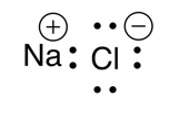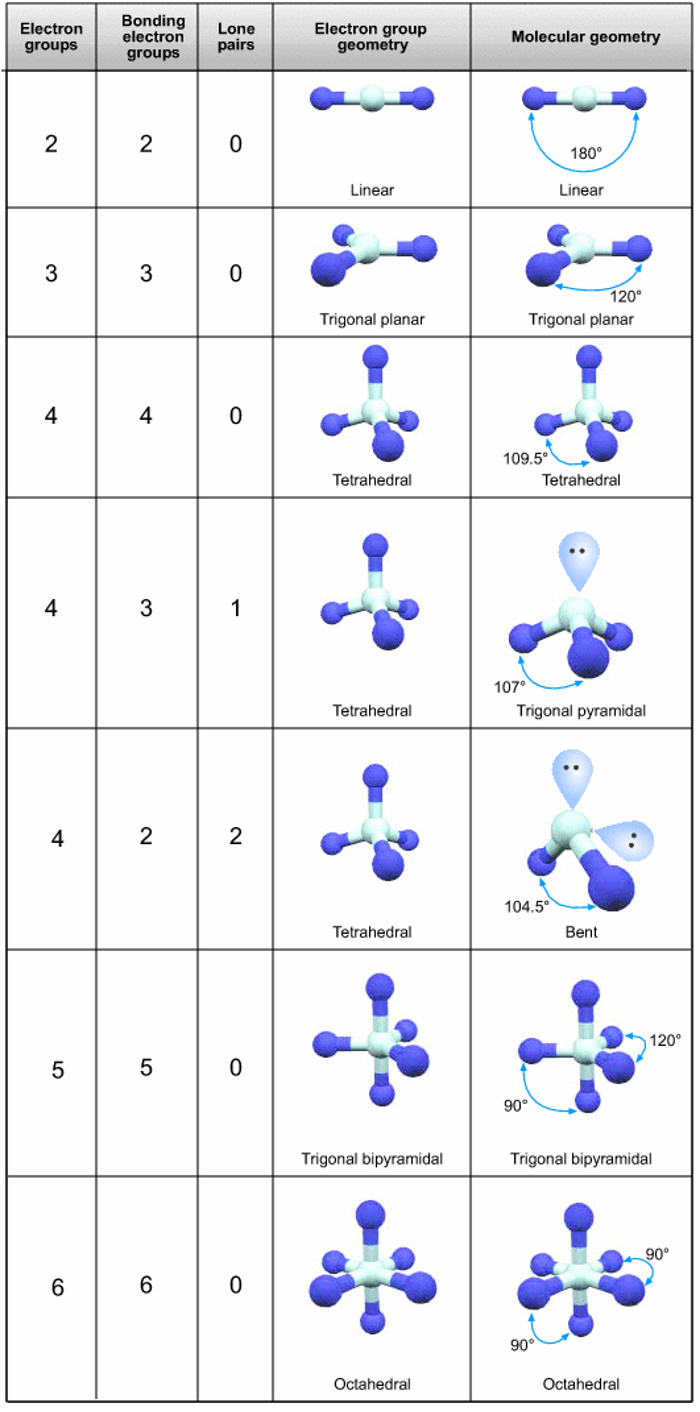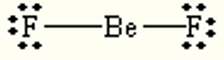# Lewis Structure & VSEPR Theory

This is part of preliminary HSC Chemistry course under the topic of Bonding

### HSC Chemistry Syllabus

• Investigate the differences between ionic and covalent compounds through:
• Using nomenclature, valency, and chemical formulae (including Lewis dot diagrams) (ACSCH029)
• Modelling the shapes of molecular substances (ACSCH056, ACSCH057)

### Lewis Structure & VSEPR Theory

A Lewis dot structure provides a graphical representation of a molecule's structure. They depict the arrangement of electrons in a compound, and students must learn to construct these structures to comprehend the electronic arrangement within compounds.

Here are the steps to create a Lewis Dot Structure:

1. Identify if a compound is covalent or ionic.

• Generally, compounds comprising a mixture of metals and non-metals are ionic. However, exceptions do exist, such as BeCl2, which is covalent due to its high ionization energy, compact size, and significant charge density.
• For covalent compounds, the structure represents the entire molecule, while for ionic compounds, each ion's structure is considered separately. One must memorize the formula and charge of any polyatomic structures within the compound.

2. Determine the total number of valence electrons available for bonding.

• Calculate the total valence electrons available for each atom in the molecule.
• Add an electron for each negative charge, and subtract an electron for each positive charge. The number of electron groups equals the number of electrons divided by 2. If the number of electrons is odd, only count the pairs.

3. Arrange the atoms with a central atom (typically the least electronegative) surrounded by outer atoms.

• Bear in mind that hydrogen is always an outer atom.

4. Assign electron pairs in the following way:

• Connect all atoms to the central atom with single bonds, each representing one shared electron pair.
• Complete octets on all outer atoms.
• If necessary, add additional electrons to the central atom or draw double bonds (you will be told if multiple bonds exist).

5. Calculate the formal charge on the central atom.

• This can be done by subtracting the number of bonding electrons and lone pair electrons from the total number of available electrons.

Example: BeF_2

1. Beryllium Fluoride is a covalent compound.

2. The total number of available electrons equals 16.

• Beryllium belongs to group 2 and thus has 2 available electrons.
• Fluorine belongs to group 17 and has 7 available electrons. With 2 Fluorines, there are 14 total available electrons for fluorine.
• Overall, there are 16 valence electrons that can be arranged.

3. The least electronegative atom is Beryllium.

F       Be        F

4. Draw in the bonds
F –– Be –– F

5. There are 12 lone pair electrons and 4 bonding electrons. The formal charge is 16-12-4 = 0.

Example: NaCl

1. Sodium Chloride is an ionic compound, so we will consider its ions.

2. The total number of available electrons equals 8.

• Sodium is in group 1 and thus has 1 available electron.
• Chlorine is in group 17 and has 7 available electrons.
• Altogether, there are 8 electrons.

3. In ionic compounds, there are only 2 ions.

Na      Cl

4. Draw the ions### Molecular Geometry of SubstancesThe three-dimensional shape of a molecule significantly influences its chemical properties and behavior but cannot be determined simply by observing its molecular formula. We are able to make informed predictions about the geometry of a particular molecule by studying its Lewis dot structure.  By studying the Lewis dot structure of a molecule, we can make informed predictions about its geometry. This is critically important, as the geometry of a molecule can profoundly affect its various properties. These properties include, but are not limited to, its polarity (which influences its solubility and reactivity), phase of matter (whether it exists as a solid, liquid, or gas under specific conditions), color, magnetic properties, and even its biological activity (for example, the efficacy of a drug molecule can be influenced by its shape). The Valence-Shell Electron-Pair Repulsion Theory (VSEPR Theory) is a model used in chemistry to predict the geometry of individual molecules based upon the extent of electron-pair electrostatic repulsion. It differentiates between 'electron group geometry' and 'molecular geometry.' Electron group geometry refers to the arrangement of all electron groups around a central atom, while molecular geometry describes the structure considering only the positioning of atoms.VSEPR Theory Basics

The key principle of the VSEPR theory is that valence electrons repel each other and will therefore strive to adopt an arrangement that maximizes the attractive forces and minimizes the repulsive forces within the molecule. As a result, electron pairs will distance themselves as far as possible to reduce repulsion. This behavior also decreases the molecule's energy, thereby enhancing its stability.

To consider the shape of the molecule, we examine two categories:

• Electron-group geometry
• Molecular geometry

Electron group geometry depends on the number of electron groups present in the molecule, leading to shapes like linear, trigonal planar, tetrahedral, and others.

Molecular geometry, while similar to electron group geometry, also considers the number of lone pairs present. The interaction between these lone pairs and bonding electrons can subtly alter the molecular geometry from the electron group geometry. Consequently, compounds like CO2 and NH3, while tetrahedral in terms of electron group geometry, exhibit bent and trigonal pyramidal molecular geometries, respectively. This deviation is also due to the influence of molecular bonding on molecular geometry."

Using VSEPR to determine the shape of a molecule:

• Draw the Lewis dot structure diagram
• E.g. Beryllium Fluoride

• Determine the total number of electrons pairs in the valence shell of the central atom.
• If we consider the lewis dot structure we can see that on the central beryllium, there are two bonds which indicate 2 electron pairs.

• Determine the number of lone pairs and position them around the central such that:
• We don’t see any lone pair electrons on it and thus there are 2 electron groups, 2 bonding electron pairs, and 0 lone pair electrons. If we look at the table, we can see that this arrangement corresponds to the linear electron group geometry and linear molecular geometry